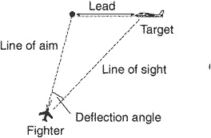# deflection angle

Also found in: Dictionary, Thesaurus, Medical.

## deflection angle

[di′flek·shən ‚aŋ·gəl]
(geodesy)
The angle at a point on the earth between the direction of a plumb line (the vertical) and the perpendicular (the normal) to the reference spheroid; this difference seldom exceeds 30 seconds of arc.
(ordnance)
An angle that measures the departure of a moving object from its directed course.
The angle of a deflection shot in gunnery, measured between the line of sight to the target and the line of sight to the aiming point.
McGraw-Hill Dictionary of Scientific & Technical Terms, 6E, Copyright © 2003 by The McGraw-Hill Companies, Inc.

## deflection angle

In surveying, a horizontal angle measured from prolongation of the preceding transit line to the next line; recorded as “right” if clockwise rotation and “left” if counterclockwise.
McGraw-Hill Dictionary of Architecture and Construction. Copyright © 2003 by McGraw-Hill Companies, Inc.

## deflection anglei. In surveying, a horizontal angle measured from the prolongation of the preceding line to the following line. Deflection angles to the right are positive; those to the left are negative.
ii. In photogrammetry, a vertical angle, measured in the vertical plane containing the flight line by which the datum of any model in a stereo-triangulated strip departs from the datum of the preceding model.
iii. In gunnery, the angle that the aircraft needs to lay ahead of the target aircraft to provide for the time of flight of the weapon to the target. It is normally measured in milliradians.
References in periodicals archive ?
So the simulation results such as the pressure field, velocity field near the bend while the projectile passing through the bend are examined to analyze the influence of the deflection angle on the projectile penetration.
In the equation, [[??].sub.i1d] is the differential of the trajectory inclination angle and trajectory deflection angle after filtering, and [k.sub.i1] = diag{[k.sub.i11], [k.sub.i12]} is the positive definite matrix.
A cursory glance at this table is enough to convince us that the unpolarized differential cross sections and, of course, the deflection angles, depend on the spin and energy of the scattered particle.
Caption: Figure 9: Comparison of elevator deflection angle with disturbance.
When the cavitator number [sigma] was constant, the stable movement of the supercavitating vehicle could be effectively achieved by the feedback control gain value adjustment of the deflection angle of fin k in the corresponding range, for the vehicle stable control to be guided.
When cavitation number [sigma] is constant, the value of feedback control gain of fin deflection angle k can be adjusted within the green stable region and the red periodic region to realize stable motion of supercavitating vehicle efficiently, which is instructive to stability control of supercavitating vehicle.
where R is curvature; CCR represents change rate of curvature; DF denotes deflection angle of curve; m, n, [a.sub.1], and [b.sub.1] are parameters.
i) When the deflection angle is parallel to the ramp wedge surface (i.e.) 15 deg.
Because the deflection angle is very small, we can assume that x = [r.sub.0]; thus on point (x, y), its coordinate can be written as ([r.sub.0], y) , then the force acted on photon reads
[phi] and 5erepresent the orientation of aircraft (pitch angle) in the earth-axis system and elevator deflection angle.
For nonstraight cracks, Tilbrook and Hoffman  employed a simple analytical model to predict mechanical energy release rate and deflection angle for a range of crack shapes under mixed-mode loading.

Site: Follow: Share:
Open / Close